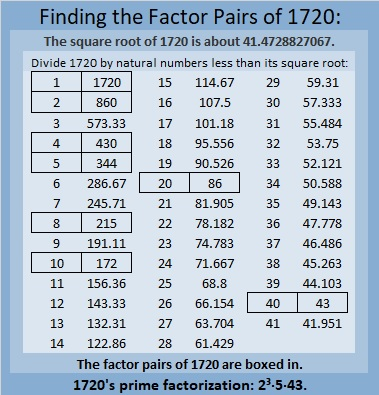# 1720 Transforming Puzzle-A-Day’s Wizard’s Hat

Contents

### Today’s Puzzle:

I recently saw a fun-to-solve puzzle from Puzzle a Day on Twitter:

The puzzle got me thinking, what would happen if the numbers weren’t from 1 to 10, but were from 0 to 9 instead? Would it change the puzzle a little or a lot?It actually changes the puzzle quite a bit. There are a lot more solutions than for the Puzzle a Day puzzle! And I don’t mean just switching the red line with the green line and/or switching the purple circles at the bottom. How many solutions can you find?

### Factors of 1720:

• 1720 is a composite number.
• Prime factorization: 1720 = 2 × 2 × 2 × 5 × 43, which can be written 1720 = 2³ × 5 × 43.
• 1720 has at least one exponent greater than 1 in its prime factorization so √1720 can be simplified. Taking the factor pair from the factor pair table below with the largest square number factor, we get √1720 = (√4)(√430) = 2√430.
• The exponents in the prime factorization are 3,1 and 1. Adding one to each exponent and multiplying we get (3 + 1)(1 + 1) (1 + 1) = 4 × 2 × 2 = 16. Therefore 1720 has exactly 16 factors.
• The factors of 1720 are outlined with their factor pair partners in the graphic below.### More About the Number 1720:

1720 is the difference of two squares in FOUR different ways:
431² – 429² = 1720,
217² – 213² = 1720,
91² – 81² = 1720, and
53² – 33² = 1720.

This site uses Akismet to reduce spam. Learn how your comment data is processed.